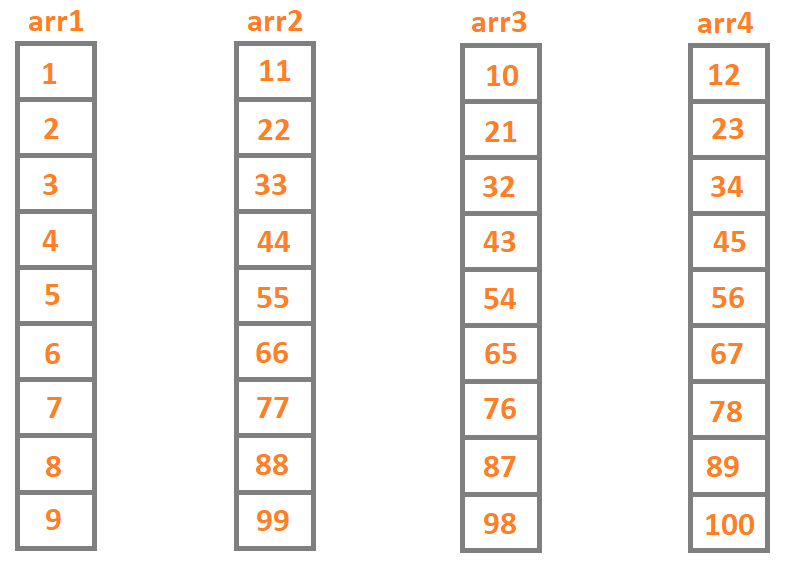# Find Nth positive number whose absolute difference of adjacent digits is at most 1

## Introduction

In this article, we will discuss the problem of finding the Nth positive number whose absolute difference of adjacent digits is at most 1. Such problems have been asked in various competitive coding platforms. The solution to this problem has many approaches with varying complexities. This article will discuss the approach having queue implementation.

Readers with no prior knowledge of queue data structure may refer to this article Queue from our CN Library.

## Problem Statement

Given a positive number N, find and display the Nth positive number whose absolute difference of adjacent digits is at most 1.

## Example

Sample_INPUT: N = 5

Expected_OUTPUT: 5

Sample_INPUT: N = 17

Expected_OUTPUT: 33

## Approach and Explanation

Absolute difference of adjacent digits is at most 1 means numbers whose difference of adjacent digits is -1, 0, or 1. Such numbers include 1 to 12, 21, 22, 23, 32, 33, 34, and so on. Our task is to find the Nth such digit.

Let us take a small example to understand the problem better.

• Consider an array arr1 having elements from 1 to 9.

• To make an array of numbers having a difference of adjacent digits as 0, we simply multiply each digit by ten and add mod ten. This array can be called arr2.

• To make an array with numbers having differences of adjacent digits as -1, we simply subtract 1 from each element of arr2. We name this array arr3.

• To make an array of numbers having a difference of adjacent digits as 1, we simply add 1 to each element of arr2. We name this array arr4.

• The cumulative sorted array formed by arr1, arr2, arr3, and arr4 is a valid solution array.

• For visual reference, the arrays formed are as follows:Now, to implement this logic, we can follow these steps:

• Check if the number is less than or equal to 12.

• If true, then print the number itself. If false, then do the following steps.

• Create an integer array of size N+1, and a new Queue.

• Enqueue the digits 1 to 9 in the queue.

• Declare a for loop from 1 to N.
• Add the number at the bottom of the queue at arr[i] and dequeue.
• In a temporary variable (say smallAns) find and store the value of arr[i]*10 + arr[i]%10. .
• If arr[i] %10 != 0, enqueue smallAns+1. (This will help make arr3, as seen in the explanation above).
• Enqueue smallAns. (This will help make arr2, as seen in the explanation above).
• If arr[i] %10 != 9, enqueue smallAns-1. (This will help make arr4, as seen in the explanation above).

• Once the loop is complete, print arr[N]

The java implementation of the given logic is given below. It is recommended to write the code first before moving to the solution code.

## Java Implementation

``````import java.util.Queue;

public class NthPosNum{

static void findNNumber(int nDigit)
{
if(nDigit <= 12){
System.out.println(nDigit);
return;
}

int[] posArr = new int[nDigit + 1];
int smallAns;

for(int i = 1; i <= 9; i++)

for(int i = 1; i <= nDigit; i++)
{
posArr[i] = queue.peek();
queue.remove();

smallAns = posArr[i] * 10 + posArr[i] % 10;
if (posArr[i] % 10 != 0){
}

if (posArr[i] % 10 != 9) {
}
}
System.out.println(posArr[nDigit]);
}

public static void main(String[] args)
{
int nDigit = 32;
findNNumber(nDigit);
}
}``````

OUTPUT

``88``

## Complexities

### Time Complexity

In the given implementation, if the value of nDigit is less than 12, then it takes O(1) time to compute. Else, we make nDigit computations to fill our array. Thus time complexity is,

T(n) = O(N),

where N is the Nth digit to be found.

### Space Complexity

In the given implementation, if the value of nDigit is less than 12, then it takes O(1) space to compute. Else, we create an array of size nDigit to store all the numbers whose absolute difference is at most 1. Thus,

Space Complexity = O(N),

where N is the Nth digit to be found.

1. What do you mean by the absolute difference of adjacent digits is at most 1?
Absolute difference of at most 1 means that the mod of difference of the two numbers should be one. The difference can be -1, 0, or 1. Thus digits such as 1, 22, 234, 345, and so on can be considered such numbers are the difference of the digits is either 0, 1, or -1.

2. What is the difference between a Stack and a Queue?
A Stack is a data structure following the LIFO (Last In First Out) order. A Queue is a data structure following the FIFO (First In First Out) order. To get a deeper idea of the two, please click Stack and Queue to find various articles covering all the concepts related to Stack and Queue.

## Key Takeaways

To summarize the article, we discussed the nth positive number whose absolute difference of adjacent digits is at most 1 problem in depth. We saw the problem statement, an example, and an approach. We also saw the Java implementation of the approach along with the time and space complexities. We summed up the article with a few FAQs.

Improve your coding skills by practising various problems of various difficulty levels on our CodeStudio.

Learn about various topics like Web Technologies, Programing Fundamentals, Aptitude, DSA, and much more from our CN Library | Free Online Coding Resources.

Happy Coding!